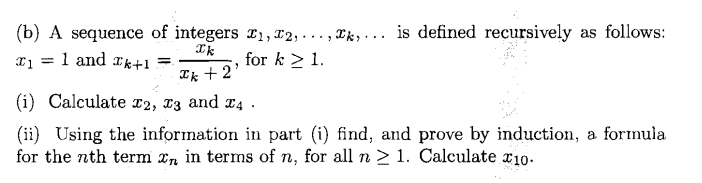# Prove by induction a formula for $x_{k+1}=\frac{x_k}{x_k+2}$, $x_1=1$

I have a IT Maths exam coming up and I just can't figure out this question. Any help would be appreciated thanks.A sequence of integers $x_1,x_2,\dots,x_k,\dots$ is defined recursively by $x_1=1$ and $x_{k+1}=\frac{x_k}{x_k+2}$, for $k\ge1$.
(i) Calculate $x_2$, $x_3$ and $x_4$.
{ii) Using the information in part (u) find, and prove by induction, a formula for the $n$the term $x_n$ in terms of $n$, for all $n\ge1$. Calculate $x_{10}$.

• I can't figure your question as well... That's two of us... You should state your problem you know... – Martigan Aug 11 '14 at 13:33
• If you're just asking how to prove something by induction, see, for example, zimmer.csufresno.edu/~larryc/proofs/proofs.mathinduction.html . – Sami Laine Aug 11 '14 at 13:38
• Further to @Martigan point, which part of the question are you having an issue with? Also, show where possible what you have tried. – Chinny84 Aug 11 '14 at 13:38

So after we work out the first values we conjecture $x_n=\frac{1}{2^n-1}$ $$\frac{1}{2^n-1}+2=\frac{1}{2^n-1}+\frac{2^{n+1}-2}{2^n-1}= \frac{2^{n+1}-1}{2^n-1}$$ $$x_{n+1}=\frac{\frac{1}{2^n-1}}{\frac{1}{2^n-1}+2}=\frac{1}{2^{n+1}-1}$$

so our conjecture is correct.

$$x_{10}=\frac{1}{2^{10}-1}=\frac{1}{1023}$$

(i) is simple enough; we have $x_1=1,x_2=\frac13,x_3=\frac17,x_4=\frac1{15}$

(ii) If you notice, the denominator for each number above is one less than a power of two. From this it would seem that $x_n=\frac1{2^n-1}$. It can be proved as follows:

Let $S_n$ be the statement $x_n=\frac1{2^n-1} \forall n\geq1$.

$S_1:x_1=\frac{1}{2^0-1}=1$

$\therefore$ $S_1$ is true. Assume $S_k$ is true for some $k\geq1$.

$S_{k+1}:x_{k+1}=\frac{x_k}{x_k+2}=\frac{\frac1{2^k-1}}{\frac1{2^k-1}+2}=\frac{1}{1+2(2^k-1)}=\frac{1}{2^{k+1}-1}$

$\therefore$ $S_{k+1}$ is true whenever $S_k$ is true.

$\therefore$ $S_n$ is true $\forall n\geq1$ $\blacksquare$

From there $x_{10}=\frac1{2^{10}-1}=\frac1{1023}$